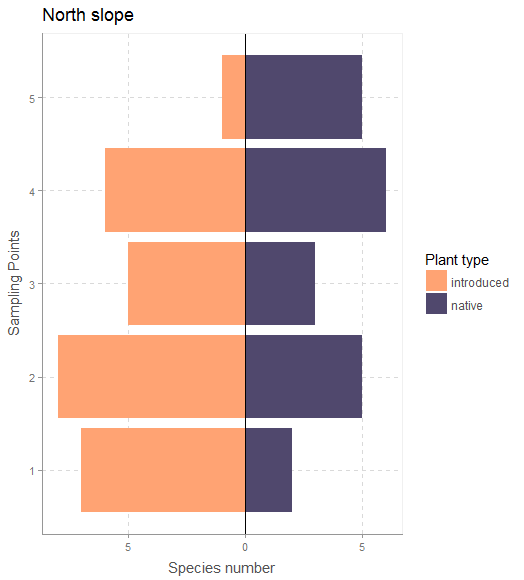Bar plots are a good way to show continuous (or count) data that is grouped into categories. When we don’t have too many categories (~4 or fewer), plotting bars side by side (dodged) is probably the most straightforward and common solution.

Here is an example for some vegetation data in which the richness of native vs. introduced plant species was measured at five sampling points along the two slopes of a ravine.

If we have only two categories and we want to show the contrast in values between the two, then diverging ‘stacked’ bar plots (thanks to data scientist Matt Sandy @appupio for the terminology) look to be a pretty effective visualization strategy.

Last month, as part of a group exercise at a workshop, we were plotting some vegetation data with only two categories. My suggestion was to plot dodged bars, but marine biologist Antonio Canepa Oneto sketched a diverging bar plot on paper and suggested we give that a try. I had never tried to make a plot like this and I couldn’t find any documentation for making such plots in R, but after a few minutes of fiddling with the stat argument in ggplot2 we were able to make a nice figure that really highlighted the differences in values between two groups.

Since then I’ve noticed these types of plots online, mainly in some journalistic figures for topics that involved very dichotomous variables (pro and versus GMO, US elections, etc.).

Here’s some R code to create stacked bar charts using ggplot2. The figure below should be fully reproducible, and it more or less follows the type of plot of plant diversity that inspired this post.

The block of code below goes through five major steps to produce the following figures:diverging bar plot with a custom theme for one of the two slopes

Both of these figures use custom themes from Bart Smeets’ artyfarty package.